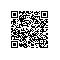# Number和Math类

1 概述 -
2 Math类 -
3 Number和Math类方法 -
4 Math的floor,round和ceil方法实例比较 -

## 1.概述

int a = 5000;
float b = 13.65f;
byte c = 0x4a;public class Test{

public static void main(String[] args){
Integer x = 5;
x =  x + 10;
System.out.println(x);
}
}

15

x 被赋为整型值时，由于 x 是一个对象，所以编译器要对x进行装箱。然后，为了使 x 能进行加运算，所以要对 x 进行拆箱。

## 2.Math类

Java 的 Math 包含了用于执行基本数学运算的属性和方法，如初等指数、对数、平方根和三角函数。

Math 的方法都被定义为 static 形式，通过 Math 类可以在主函数中直接调用。

public class Test {
public static void main (String []args)
{
System.out.println("90 度的正弦值：" + Math.sin(Math.PI/2));
System.out.println("0度的余弦值：" + Math.cos(0));
System.out.println("60度的正切值：" + Math.tan(Math.PI/3));
System.out.println("1的反正切值： " + Math.atan(1));
System.out.println("π/2的角度值：" + Math.toDegrees(Math.PI/2));
System.out.println(Math.PI);
}
}

90 度的正弦值：1.0
0度的余弦值：1.0
60度的正切值：1.7320508075688767
1的反正切值： 0.7853981633974483
π/2的角度值：90.0
3.141592653589793

Number和Math类方法

## 3.Number和Math类方法

1 xxxValue() 将 Number 对象转换为xxx数据类型的值并返回。
2 compareTo() 将number对象与参数比较。
3 equals() 判断number对象是否与参数相等。
4 valueOf()返回一个 Number 对象指定的内置数据类型
5 toString() 以字符串形式返回值。
6 parseInt() 将字符串解析为 int 类型。
7 abs() 返回参数的绝对值。
8 ceil() 返回大于等于( >= )给定参数的的最小整数，类型为双精度浮点型。
9 floor() 返回小于等于（ <= ）给定参数的最大整数 。
10 rint() 返回与参数最接近的整数。返回类型为 double。
11 round() 它表示四舍五入，算法为 Math.floor(x+0.5)，即将原来的数字加上 0.5 后再向下取整，所以，Math.round(11.5) 的结果为12，Math.round(-11.5) 的结果为-11。
12 min() 返回两个参数中的最小值。
13 max() 返回两个参数中的最大值。
14 exp() 返回自然数底数e的参数次方。
15 log() 返回参数的自然数底数的对数值。
16 pow() 返回第一个参数的第二个参数次方。
17 sqrt() 求参数的算术平方根。
18 sin() 求指定double类型参数的正弦值。
19 cos() 求指定double类型参数的余弦值。
20 tan() 求指定double类型参数的正切值。
21 asin() 求指定double类型参数的反正弦值。
22 acos() 求指定double类型参数的反余弦值。
23 atan() 求指定double类型参数的反正切值。
24 atan2() 将笛卡尔坐标转换为极坐标，并返回极坐标的角度值。
25 toDegrees() 将参数转化为角度。
26 toRadians() 将角度转换为弧度。
27 random() 返回一个随机数。

## 4.Math的floor,round和ceil方法实例比较

1.4 1 1 2
1.5 1 2 2
1.6 1 2 2
-1.4 -2 -1 -1
-1.5 -2 -1 -1
-1.6 -2 -2 -1

floor, roundceil 实例：

public class Main {
public static void main(String[] args) {
double[] nums = { 1.4, 1.5, 1.6, -1.4, -1.5, -1.6 };
for (double num : nums) {
test(num);
}
}

private static void test(double num) {
System.out.println("Math.floor(" + num + ")=" + Math.floor(num));
System.out.println("Math.round(" + num + ")=" + Math.round(num));
System.out.println("Math.ceil(" + num + ")=" + Math.ceil(num));
}
}

Math.floor(1.4)=1.0
Math.round(1.4)=1
Math.ceil(1.4)=2.0
Math.floor(1.5)=1.0
Math.round(1.5)=2
Math.ceil(1.5)=2.0
Math.floor(1.6)=1.0
Math.round(1.6)=2
Math.ceil(1.6)=2.0
Math.floor(-1.4)=-2.0
Math.round(-1.4)=-1
Math.ceil(-1.4)=-1.0
Math.floor(-1.5)=-2.0
Math.round(-1.5)=-1
Math.ceil(-1.5)=-1.0
Math.floor(-1.6)=-2.0
Math.round(-1.6)=-2
Math.ceil(-1.6)=-1.0